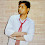# VBA : For - Next Loop

This tutorial will show you how to use FOR NEXT loop in VBA.

What is loop ?

It allows you to execute code repeatedly until a certain condition is reached.

The syntax for FOR NEXT loop is as follows :
For < Counter Variable>= <Initial Value> To <End Value>
<Code that you want to repeat through loop>
Next <Counter Variable>
The example is shown in the image below :

Examples

1. Write series of numbers

The following code writes values from 1 to 5 in column A :
Sub forloop()
Dim i As Integer
For i = 1 To 5
Range("A" & i).Value = i
Next i
End Sub
In the first loop counter, Range("A" & i) evaluates to Range("A"&1) i.e. Range("A1"). In the second loop counter, Range("A" & i) evaluates to Range("A"&2) i.e. Range("A2"). Then the range increments by 1 till fifth row.

The output is shown in the image below :

2. Multiply column C values by 2

The data is shown in the image below :

Sub Dynamic()
Dim last As Double, i As Integer
With ActiveSheet
last = .Cells(.Rows.Count, "C").End(xlUp).Row - 2
End With
For i = 1 To last
Range("D" & i + 2).Formula = Range("C" & i + 2).Value * 2
Next i
End Sub
Explanation :

1.  The following code identifies the last used row in column C. 2 is substracted from the code because column C contains blank row and header at the beginning of the column.

With ActiveSheet
last = .Cells(.Rows.Count, "C").End(xlUp).Row - 2
End With

2. In the first loop counter, Range("D" & i + 2) evaluates to Range("D"&1+2) i.e. Range("D3"). Then the range increments by 1 till last used row.

3. Filling a column with consecutive numbers in consecutive cells

You can increment counter variable by any value you like. The Step keyword tells excel to increment counter variable by the specified value.
Sub forloopstep()
Dim i As Integer
For i = 1 To 10 Step 2
Range("A" & i).Value = i
Next i
End Sub
The Step 2 code tells excel to increment i each time through the loop by 2.

The output is shown in the image below :

Related Posts
ShareDeepanshu founded ListenData with a simple objective - Make analytics easy to understand and follow. He has over 10 years of experience in data science. During his tenure, he worked with global clients in various domains like Banking, Insurance, Private Equity, Telecom and HR.

1 Response to "VBA : For - Next Loop"
1.Greetings,

I have a excel sheet for which i want a VBA code to merge 10 consecutive rows in a column and repeating it till the end of rows. So after every 10 rows the next 10 rows gets automatically merged.

i would be really thankful if any help on this task is provided.

Next → ← Prev
Looks like you are using an ad blocker!

To continue reading you need to turnoff adblocker and refresh the page. We rely on advertising to help fund our site. Please whitelist us if you enjoy our content.